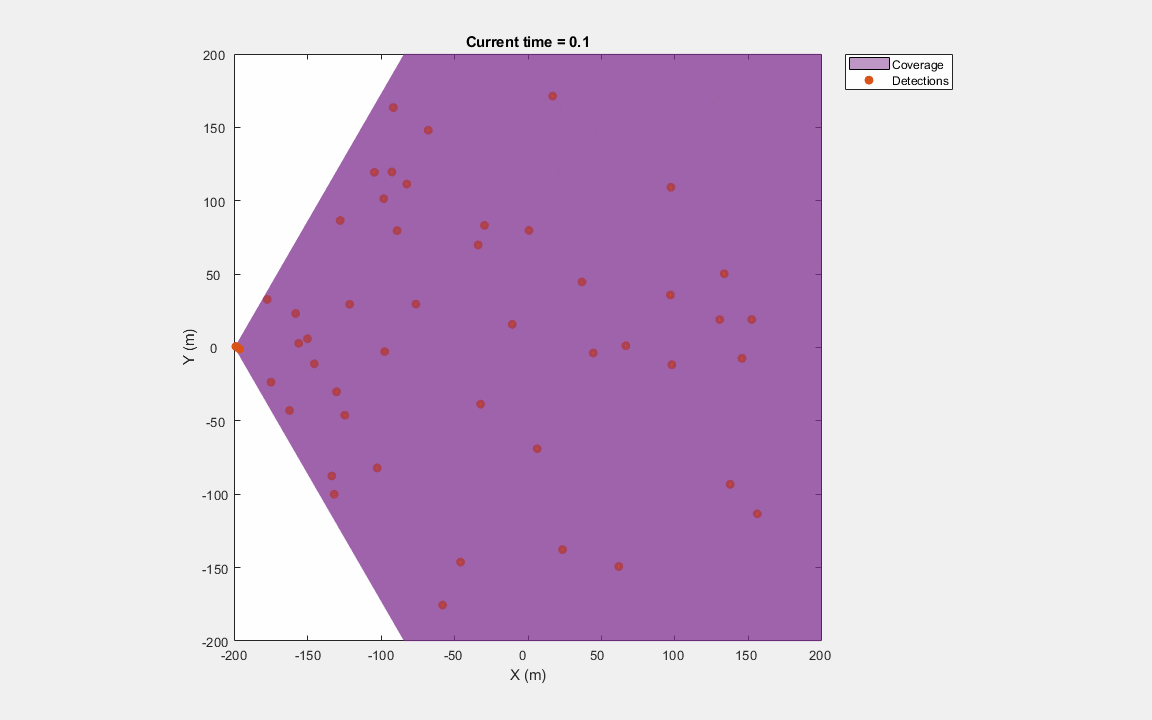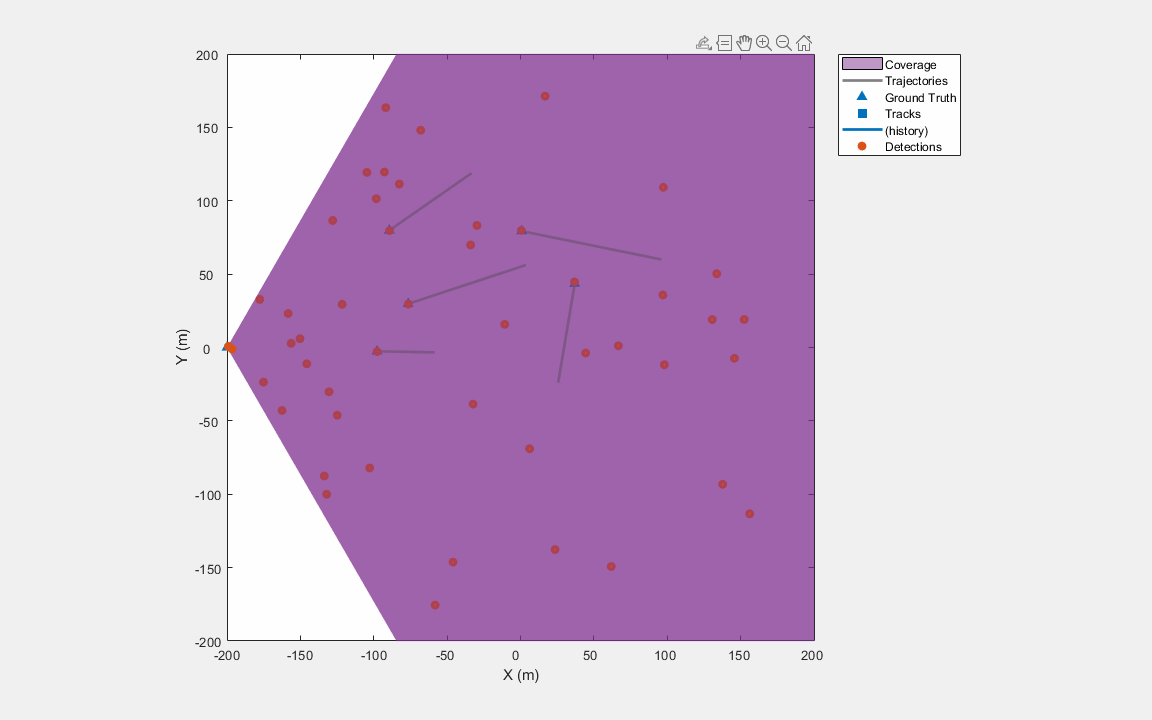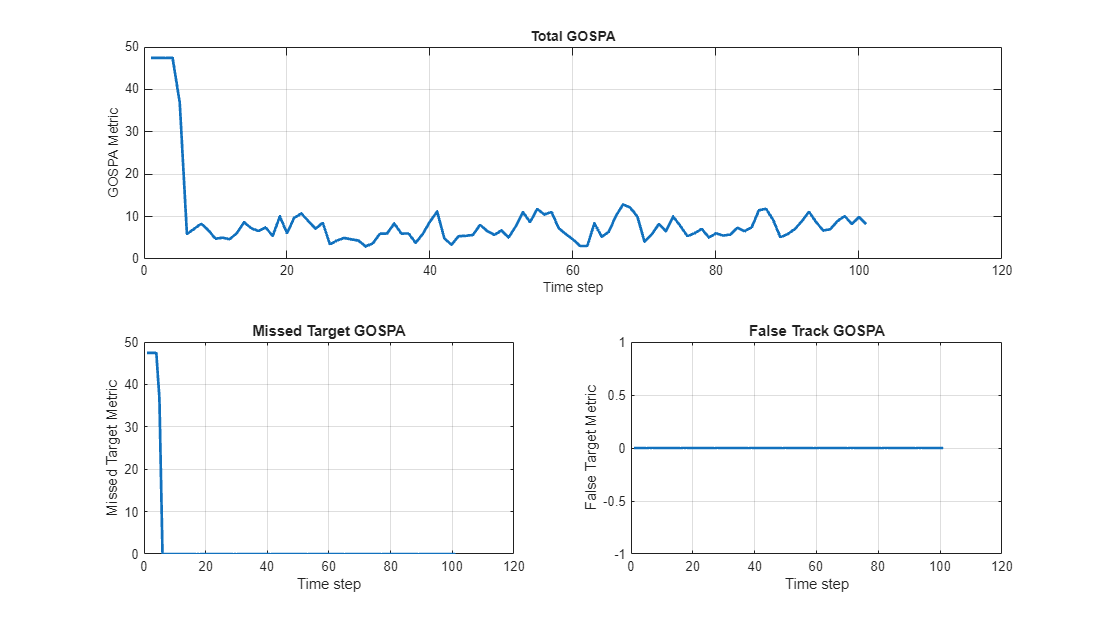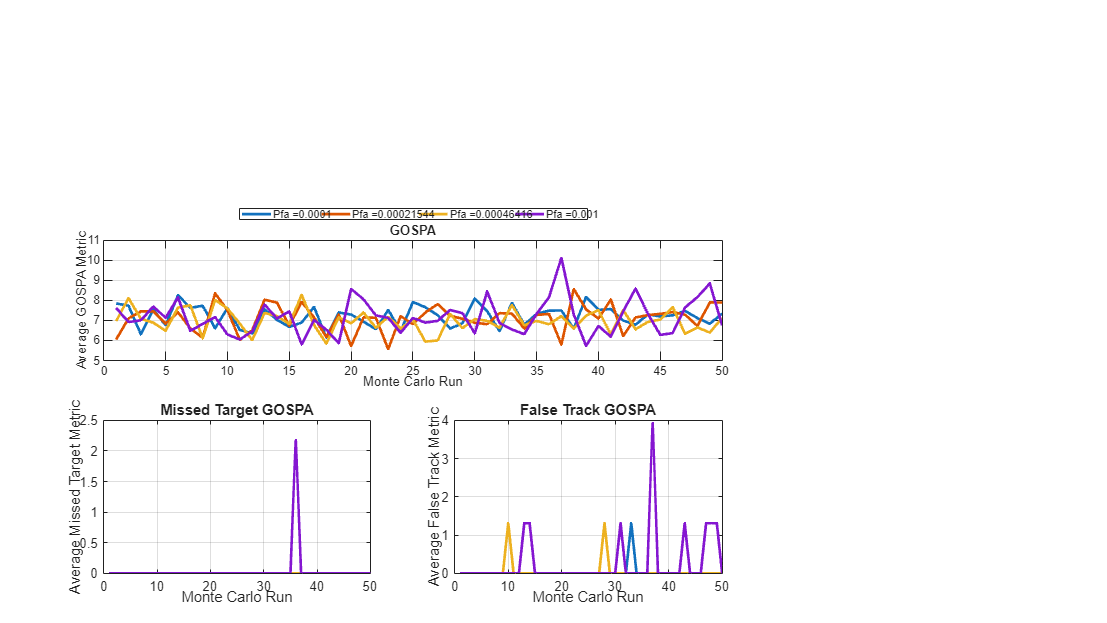# Track Point Targets in Dense Clutter Using GM-PHD Tracker

This example shows you how to track points targets in dense clutter using a Gaussian mixture probability hypothesis density (GM-PHD) tracker using a constant velocity model.

### Setup Scenario

The scenario used in this example is created using `trackingScenario`. The scenario consists of five point targets moving at a constant velocity. The targets move within the field of view of a static 2-D radar sensor. You use the `monostaticRadarSensor` to simulate the 2-D sensor and mount it on a static platform. You use the `FalseAlarmRate` property of the sensor to control the density of the clutter. The value of the `FalseAlarmRate` property represents the probability of generating a false alarm in one resolution cell of the sensor. Based on a false alarm rate of ${10}^{-3\text{\hspace{0.17em}}}$and the resolution of the sensor defined in this example, there are approximately 48 false alarms generated per step.

```% Reproducible target locations rng(2022); % Create a trackingScenario object scene = trackingScenario('UpdateRate',10,'StopTime',10); numTgts = 5; % Initialize position and velocity of each target x = 100*(2*rand(numTgts,1) - 1); y = 100*(2*rand(numTgts,1) - 1); z = zeros(numTgts,1); vx = 5*randn(numTgts,1); vy = 5*randn(numTgts,1); vz = zeros(numTgts,1); % Add platforms to scenario with given positions and velocities. for i = 1:numTgts thisTgt = platform(scene); thisTgt.Trajectory.Position = [x(i) y(i) z(i)]; thisTgt.Trajectory.Velocity = [vx(i) vy(i) vz(i)]; end % Add a detecting platform to the scene. detectingPlatform = platform(scene); detectingPlatform.Trajectory.Position = [-200 0 0]; % Simulate 2-D radar using a monostaticRadarSensor. radar = monostaticRadarSensor(1,... 'UpdateRate',scene.UpdateRate,... 'DetectionProbability',0.9,...% Pd 'FalseAlarmRate',1e-3,...% Pfa 'FieldOfView',[120 1],... 'ScanMode','No Scanning',... 'DetectionCoordinates','scenario',...% Report in scenario frame 'HasINS',true,... % Enable INS to report in scenario frame 'ReferenceRange',300,... % Short-range 'ReferenceRCS',10,... % Default RCS of platforms 'MaxUnambiguousRange',400,... % Short-range 'RangeResolution',1,... 'AzimuthResolution',1,... 'HasFalseAlarms',true); % Reports false alarms % Mount the radar on the detecting platform. detectingPlatform.Sensors = radar;```### Set Up Tracker and Metrics

#### Tracker

You use a GM-PHD point object tracker to track targets. The first step towards configuring a PHD tracker is to set up the configuration of the sensor. You define the configuration using a `trackingSensorConfiguration` object.

The `SensorIndex` of the configuration is set to 1 to match that of the simulated sensor. As the sensor is a point object sensor that outputs at most one detection per object per scan, you set the `MaxNumDetsPerObject` property of the configuration to 1.

The `SensorTransformFcn`, `SensorTransformParameters,` and `SensorLimits` together allow you to define the region in which the tracks can be detected by the sensor. The `SensorTransformFcn` defines the transformation of a track state (${\mathit{x}}_{\mathrm{track}}$) into an intermediate space used by the sensor (${\mathit{x}}_{\mathrm{sensor}}$) to define track detectability. The overall calculation to calculate detection probability is shown below:

`${\mathit{x}}_{\mathrm{sensor}}\text{\hspace{0.17em}}=\mathrm{SensorTransformFcn}\left({\mathit{x}}_{\mathrm{track}},\text{\hspace{0.17em}}\mathrm{SensorTransformParameters}\right)$`

`${\mathit{P}}_{\mathit{d}\text{\hspace{0.17em}}}=\text{\hspace{0.17em}}$`

`$\left\{\begin{array}{ll}\mathrm{Configuration}.\mathrm{DetectionProbability}& {\mathrm{SensorLimits}\left(:,1\right)\text{\hspace{0.17em}}\le \text{\hspace{0.17em}}\mathit{x}}_{\mathrm{sensor}}\le \mathrm{SensorLimits}\left(:,2\right)\\ \mathrm{Configuration}.\mathrm{MinDetectionProbability}& \mathrm{otherwise}\end{array}$`

To compute detection probability of an uncertain state with a given state covariance, the tracker generates samples of the state using sigma-point calculations similar to an unscented Kalman filter.

Notice that the signature of the `SensorTransformFcn` is similar to a typical measurement model. Therefore, you can use functions like `cvmeas`, `cameas` as `SensorTransformFcn`. In this example, you assume that all tracks are detectable. Therefore, the `SensorTransformFcn` is defined as `@(x,params)x` and SensorLimits are defined as `[-inf inf] `for all states.

```% Transform function and limits sensorTransformFcn = @(x,params)x; sensorTransformParameters = struct; sensorLimits = [-inf inf].*ones(6,1); % Detection probability for sensor configuration Pd = radar.DetectionProbability; config = trackingSensorConfiguration('SensorIndex',1,... 'IsValidTime',true,...% Update every step 'MaxNumDetsPerObject',1,... 'SensorTransformFcn',sensorTransformFcn,... 'SensorTransformParameters',sensorTransformParameters,... 'SensorLimits',sensorLimits,... 'DetectionProbability',Pd);```

The `ClutterDensity` property of the configuration refers to false alarm rate per-unit volume of the measurement-space. In this example, the measurement-space is defined as the Cartesian coordinates as the detections are reported in the scenario frame. As the volume of the sensor's resolution in Cartesian coordinates changes with azimuth and range of the resolution, an approximate value can be computed at the mean-range of the sensor.

```% Sensor Parameters to calculate Volume of the resolution bin Rm = radar.MaxUnambiguousRange/2; % mean -range dTheta = radar.ElevationResolution; % Bias fractions reduce the "effective" resolution size dPhi = radar.AzimuthBiasFraction*radar.AzimuthResolution; dR = radar.RangeBiasFraction*radar.RangeResolution; % Cell volume VCell = 2*((Rm+dR)^3 - Rm^3)/3*(sind(dTheta/2))*deg2rad(dPhi); % False alarm rate Pfa = radar.FalseAlarmRate; % Define clutter density config.ClutterDensity = Pfa/VCell;```

You also define a `FilterInitializationFcn` to specify the type of filter and the distribution of components in the filter, initialized by this sensor. In this example, you set the `FilterInitializationFcn` to `initcvgmphd`, which creates a constant-velocity GM-PHD filter and adds 1 component per low-likelihood detection from the tracker. The `initcvgmphd` does not add any component when called without a detection. This means that under this configuration, the birth components are only added to the filter when detections fall outside of the `AssignmentThreshold` of multi-target filer. See A`ssignmentThreshold `property of` trackerPHD `for more details.

`config.FilterInitializationFcn = @initcvgmphd;`

Next you create the tracker using this configuration using the `trackerPHD` System object™. While configuring a tracker, you specify the `BirthRate` property to define the number of targets appearing in the field of view per unit time. The `FilterInitializationFcn` used with the configuration adds one component per unassigned detection. At each time-step, you can expect the number of components to be approximately equal to the number of false alarms and new targets. The tracker distributes the `BirthRate `to all these components uniformly.

```% Use number of radar cells to compute number of false alarms per unit time. NCells = radar.MaxUnambiguousRange/radar.RangeResolution*radar.FieldOfView(1)/radar.AzimuthResolution; numFalse = Pfa*NCells; % Choose an initial weight of 0.05 for each new component. As number of new % targets is not known, new number of components are simply used as number % of false alarms. 0.1 is the update rate. birthRate = 0.05*(numFalse)/0.1; % Create a tracker with the defined sensor configuration. tracker = trackerPHD('BirthRate',birthRate,... 'SensorConfigurations', config);```

#### Metrics

To evaluate the performance of the tracker, you also set up a metric for performance evaluation. In this example, you use the Generalized Optimal Subpattern Assignment (GOSPA) metric. The GOSPA metric aims to evaluate the performance of a tracker by assigning it a single cost value. The better the tracking performance, the lower the GOSPA cost. A value of zero represents perfect tracking.

```% Create gospa metric object. gospa = trackGOSPAMetric; % Initialize variable for storing metric at each step. gospaMetric = zeros(0,1); loc = zeros(0,1); mt = zeros(0,1); ft = zeros(0,1);```

### Run Simulation

Next, you advance the scenario, collect detections from the scenario, and run the PHD tracker on the simulated detections.

```% Create a display display = helperClutterTrackingDisplay(scene); count = 1; % Counter for storing metric data rng(2018); % Reproducible run while advance(scene) % Current time time = scene.SimulationTime; % Current detections detections = detect(scene); % Tracks tracks = tracker(detections, time); % Update display display(scene, detections, tracks); % Compute GOSPA. getTruth function is defined below. truths = getTruth(scene); [~,gospaMetric(count),~,loc(count),mt(count),ft(count)] = gospa(tracks, truths); count = count + 1; end```You can visualize that all targets were tracked by the PHD tracker in this scenario. This can also be quantitatively evaluated using the GOSPA metric and associated components. In the figure below, notice that GOSPA metric goes down after a few steps. The initial value of GOSPA metric is higher because of establishment delay for each track.

```figure('Units','normalized','Position',[0.1 0.1 0.8 0.8]) subplot(2,2,[1 2]); plot(gospaMetric,'LineWidth',2); title('Total GOSPA'); ylabel('GOSPA Metric'); xlabel('Time step'); grid on; subplot(2,2,3); plot(mt,'LineWidth',2); title('Missed Target GOSPA'); ylabel('Missed Target Metric'); xlabel('Time step'); grid on; subplot(2,2,4); plot(ft,'LineWidth',2); title('False Track GOSPA'); ylabel('False Target Metric'); xlabel('Time step'); grid on;```### Analyze Performance

A typical method to qualify the performance of a tracker is by running several simulations on different realizations of the scenario. Monte Carlo simulations help to nullify the effect of random events such as locations of false alarms, events of target misses, and noise in measurements.

In this section, you run different realizations of the scenario and the tracker with different false alarm rates and compute the average GOSPA of the system for each realization. The process of running a scenario and computing the average GOSPA for the system is wrapped inside the helper function helperRunMonteCarloAnalysis`. `To accelerate the Monte Carlo simulations, you can generate code for the `monostaticRadarSensor` model as well as the `trackerPHD `using MATLAB® Coder™ Toolbox. The process for generating code is wrapped inside the helper function helperGenerateCode. To generate code for an algorithm, you assemble the code into a standalone function. This function is named `clutterSimTracker_kernel` in this example. The `clutterSimTracker_kernel` function is written to support four false alarm rates. To regenerate code with different false alarm rates, you can use the following command.

```Pfas = 10.^(-4,-3,4); % Choose your false alarm settings helperGenerateCode(scene, Pfas); % Generate code ```

To regenerate code for more false alarm settings, you can modify the functions to include more persistent variables. For more details on how to generate code for System objects™, refer to the How to Generate C Code for a Tracker example.

The `helperRunMonteCarloAnalysis` function uses a `parfor` to execute each Monte Carlo run. If you have Parallel Computing Toolbox™ license, the Monte Carlo runs can be distributed across several workers to further accelerate the simulations.

```% Turn on flag to run Monte Carlo Simulations Pfas = 10.^linspace(-4,-3,4); runMonteCarlo = false; if runMonteCarlo numRunsPerPfa = 50; %#ok<UNRCH> gospaMCs = zeros(4,numRunsPerPfa,4); for i = 1:4 gospaMCs(i,:,:) = helperRunMonteCarloAnalysis(scene, Pfas, Pfas(i), numRunsPerPfa); end save clutterTrackingMCRuns.mat gospaMCs; else % Reload results from last run load('clutterTrackingMCRuns.mat','gospaMCs'); end % Plot results figure('Units','normalized','Position',[0.1 0.1 0.8 0.8]) subplot(2,2,[1 2]); plot(gospaMCs(:,:,1)','LineWidth',2); title('GOSPA'); legend(strcat('Pfa = ',string(Pfas)),'Orientation','horizontal','Location','NorthOutside'); ylabel('Average GOSPA Metric'); xlabel('Monte Carlo Run'); grid on; subplot(2,2,3); plot(gospaMCs(:,:,3)','LineWidth',2); title('Missed Target GOSPA'); ylabel('Average Missed Target Metric'); xlabel('Monte Carlo Run'); grid on; subplot(2,2,4); plot(gospaMCs(:,:,4)','LineWidth',2); title('False Track GOSPA'); ylabel('Average False Track Metric'); xlabel('Monte Carlo Run'); grid on;```The plots above show performance of the tracker in this scenario by running 50 Monte Carlo realizations for each false alarm rate. As the false alarm rate increases, the probability of generating a false track increases. This probability is even higher in the vicinity of the sensor, where density of resolution cells is much higher. As false alarms are closely spaced and appear frequently in this region, they can be misclassified as a low-velocity false-track. This behavior of the tracker can be observed in the averaged "False Track Component" of the GOSPA metric per scenario run. Notice that as the false alarm rate increases, the number of peaks in the plot also increase. This also causes an increase in the total GOSPA metric. The "Missed Target Component" is zero for all except one run. This type of event is caused by multiple misses of the target by the sensor.

### Summary

In this example, you learned how to configure and initialize a GM-PHD tracker to track point targets for a given false alarm rate. You also learned how to evaluate the performance of the tracker using the GOSPA metric and its associated components. In addition, you learned how to run several realizations of the scenario under different false alarm settings to qualify the performance characteristics of the tracker.

### Utility Functions

#### `helperRunMonteCarloAnalysis`

```function gospaMC = helperRunMonteCarloAnalysis(scene, Pfas, Pfai, numRuns) clear clutterSimTracker_kernel; % Compute which tracker to run based on provided Pfa settingToRun = find(Pfas == Pfai,1,'first'); % Initialize ospa for each Monte Carlo run gospaMC = zeros(numRuns,4); % Use parfor for parallel simulations parfor i = 1:numRuns rng(i, 'twister'); % Restart scenario before each run restart(scene); % metrics vs time in this scenario gospaMetric = zeros(0,4); % Counter count = 0; % Advance scene and run while advance(scene) count = count + 1; % Use radar sensor using targetPoses function own = scene.Platforms{end}; tgtPoses = targetPoses(own,'rotmat'); insPose = pose(own); poses = platformPoses(scene,'rotmat'); truths = poses(1:end-1); time = scene.SimulationTime; % Reset tracker at the end of step so at the next call tracker is % reset to time 0. systemToReset = settingToRun*(abs(time - scene.StopTime) < 1e-3); % Store ospa metric. Tracks and detections can be outputted to run % scenario in code generation without needing Monte Carlo runs. [~,~,gospaMetric(count,:)] = clutterSimTracker_kernel(Pfas, tgtPoses, insPose, truths, time, settingToRun, systemToReset); end % Compute average of GOSPA in this run % Start from time step 20 to allow track establishment. gospaMC(i,:) = mean(gospaMetric(20:end,:)); end end```

#### `helperGenerateCode`

```function helperGenerateCode(scene,Pfas) %#ok<DEFNU> % Generate sample pose using platformPoses poses = platformPoses(scene,'rotmat'); % Generate sample inputs for the kernel function % % Pfas must be compile-time constant and they cannot change with time Pfas = coder.Constant(Pfas); % Target poses as a variable size arrray of max 20 elements tgtPoses = coder.typeof(poses(1),[20 1],[1 0]); % Scalar ins pose insPose = pose(scene.Platforms{1},'true'); % Truth information with maximum 20 targets truths = coder.typeof(poses(1),[20 1],[1 0]); % Time time = 0; % Which false-alarm setting to run and which to reset. Reset is necessary % after each run to reinitialize the tracker systemToRun = 1; systemToReset = 1; inputs = {Pfas, tgtPoses, insPose, truths, time, systemToRun, systemToReset}; %#ok<NASGU> % Same name as MATLAB file allows to shadow the MATLAB function when % MEX file is available and execute code in MEX automatically. codegen clutterSimTracker_kernel -args inputs -o clutterSimTracker_kernel; end```

#### `getTruth`

```function truths = getTruth(scenario) platPoses = platformPoses(scenario); % True Information truths = platPoses(1:end-1); % Last object is ownship end```

#### `clutterSimTracker_kernel`

This function is defined in an external file named `clutterSimTracker_kernel`, available in the same working folder as this script.

```function [detections, tracks, ospaMetric] = clutterSimTracker_kernel(Pfas, tgtPoses, insPose, truths, time, systemToRun, systemToReset) assert(numel(Pfas) == 4,'Only 4 false alarm settings supported. Rewrite more persistent variables to add more settings'); persistent tracker1 tracker2 tracker3 tracker4 ... radar1 radar2 radar3 radar4 .... reset1 reset2 reset3 reset4 .... gospa if isempty(gospa) || isempty(reset1) || isempty(reset2) || isempty(reset3) || isempty(reset4) gospa = trackGOSPAMetric('CutoffDistance',50); end if isempty(tracker1) || isempty(radar1) || isempty(reset1) tracker1 = setupTracker(Pfas(1)); radar1 = setupRadar(Pfas(1)); reset1 = zeros(1,1); end if isempty(tracker2) || isempty(radar2) || isempty(reset2) tracker2 = setupTracker(Pfas(2)); radar2 = setupRadar(Pfas(2)); reset2 = zeros(1,1); end if isempty(tracker3) || isempty(radar3) || isempty(reset3) tracker3 = setupTracker(Pfas(3)); radar3 = setupRadar(Pfas(3)); reset3 = zeros(1,1); end if isempty(tracker4) || isempty(radar4) || isempty(reset4) tracker4 = setupTracker(Pfas(4)); radar4 = setupRadar(Pfas(4)); reset4 = zeros(1,1); end switch systemToRun case 1 detections = radar1(tgtPoses, insPose, time); tracks = tracker1(detections, time); case 2 detections = radar2(tgtPoses, insPose, time); tracks = tracker2(detections, time); case 3 detections = radar3(tgtPoses, insPose, time); tracks = tracker3(detections, time); case 4 detections = radar4(tgtPoses, insPose, time); tracks = tracker4(detections, time); otherwise error('Idx out of bounds'); end [~,gp,~,loc,mT,fT] = gospa(tracks, truths); ospaMetric = [gp loc mT fT]; switch systemToReset case 1 reset1 = zeros(0,1); case 2 reset2 = zeros(0,1); case 3 reset3 = zeros(0,1); case 4 reset4 = zeros(0,1); end end function tracker = setupTracker(Pfa) Pd = 0.9; VCell = 0.0609; % Inlined value of cell volume; Kc = Pfa/VCell; % Clutter density % Birth rate birthRate = 0.05*(5 + Pfa*48000)/0.1; % Transform function and limits sensorTransformFcn = @(x,params)x; sensorTransformParameters = struct; sensorLimits = bsxfun(@times,[-inf inf],ones(6,1)); % Assemble information into a trackingSensorConfiguration config = trackingSensorConfiguration('SensorIndex',1,... 'IsValidTime',true,...% Update every step 'DetectionProbability',Pd,...% Detection Probability of detectable states 'ClutterDensity',Kc,...% Clutter Density 'SensorTransformFcn',sensorTransformFcn,... 'SensorTransformParameters',sensorTransformParameters,... 'SensorLimits',sensorLimits,... 'MaxNumDetsPerObject',1,... 'FilterInitializationFcn',@initcvgmphd); tracker = trackerPHD('SensorConfigurations', config,... 'BirthRate',birthRate); end function radar = setupRadar(Pfa) Pd = 0.9; radar = monostaticRadarSensor(1,... 'UpdateRate',10,... 'DetectionProbability',Pd,... 'FalseAlarmRate',Pfa,... 'FieldOfView',[120 1],... 'ScanMode','No Scanning',... 'DetectionCoordinates','Scenario',...% Report in scenario frame 'HasINS',true,...% Enable INS to report in scenario frame 'ReferenceRange',300,...% Short-range 'ReferenceRCS',10,... 'MaxUnambiguousRange',400,...% Short-range 'RangeResolution',1,... 'AzimuthResolution',1,... 'HasFalseAlarms',true); end ```